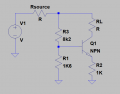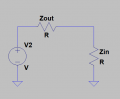# Current source having a good ratio output impedance/input impedance

#### leo0001

Joined Sep 30, 2018
79
Hi,

Here is my circuit :If I want to have a good current source of 1 mA (suppose VBE is equal to 0V6 and it is constant), I need to have 1V6 on VB.

For this i need to have a good ratio between Zout and Zin. Zout << ZinZin is equal to (B+1)RE by computing it. And it's pretty bad because it depends on B (beta) which decreases during the transistor life. So Zin will decrease... and by the way Vb will decrease ... And my current will be lower than 1 mA. In order to reduce the problem i need to have Zout largely inferior to Zin. In this case, VB will decrease more slowly !For me Zout is equal to Rsource + R3. But If i m looking an example on Internet, the output impedance is approximately equal to around R3//R1 = 1k3. I can't understand it... It doesn't make sense ... For me this output impedance is the output impedance of the emmitter follower. And it is just necessary to compute it if I want to put another stage after my current source ...

Did I make a mistake ?

Thank you,

Have a nice day !